# Samacheer Kalvi Books: Tamilnadu State Board Text Books Solutions

## Samacheer Kalvi 12th Computer Applications Chapter 7 Looping Structure Notes PDF Download: Tamil Nadu STD 12th Computer Applications Chapter 7 Looping Structure NotesSamacheer Kalvi 12th Computer Applications Chapter 7 Looping Structure Notes PDF Download: Tamil Nadu STD 12th Computer Applications Chapter 7 Looping Structure Notes

## Samacheer Kalvi 12th Computer Applications Chapter 7 Looping Structure Notes PDF Download

We bring to you specially curated Samacheer Kalvi 12th Computer Applications Chapter 7 Looping Structure Notes PDF which have been prepared by our subject experts after carefully following the trend of the exam in the last few years. The notes will not only serve for revision purposes, but also will have several cuts and easy methods to go about a difficult problem.

 Board Tamilnadu Board Study Material Notes Class Samacheer Kalvi 12th Computer Applications Subject 12th Computer Applications Chapter Chapter 7 Looping Structure Format PDF Provider Samacheer Kalvi Books

## How to Download Samacheer Kalvi 12th Computer Applications Chapter 7 Looping Structure Notes PDFs?

2. Click on the Samacheer Kalvi 12th Computer Applications Notes PDF.
3. Look for your preferred subject.
4. Now download the Samacheer Kalvi 12th Computer Applications Chapter 7 Looping Structure notes PDF.

## Download Samacheer Kalvi 12th Computer Applications Chapter 7 Looping Structure Chapterwise Notes PDF

PART – I

Question 1.
Most complicated looping structure is ……………………………
(a) While
(b) Do While
(c) For
(d) None of them
(c) For

Question 2.
Loops that iterate for fixed number of times is called ………………………….
(a) Unbounded loops
(b) Bounded loops
(c) While loops
(d) For loops
(b) Bounded loops

Question 3.
Which loop evaluates condition expression as Boolean, if it is true, it executes statements and when it is false it will terminate?
(a) For loop
(b) For each loop
(c) While loop
(d) All of them
(d) All of them

Question 4.
Which loop evaluates condition expression as Boolean, if it is true, it executes statements and when it is false it will terminate?
(a) For loop
(b) For each loop
(c) While loop
(d) All of them
(d) All of them

Question 5.
What will be displayed in a browser when the following PHP code is executed:
<?php
for (Scounter = 20; \$counter< 10;
\$counter++)
{
}
echo “Counter is: Scounter”;
?>
(b) Counter is: 20
(c) Welcome to Tamilnadu Counter is: 22
(b) Counter is: 20

Question 6.
What will be displayed in a browser when the following PHP code is executed:
<?php
for (Scounter = 10; Scounter = 10;
\$counter = \$counter + 5){
echo “Hello”;
}
?>
(a) Hello Hello Hello Hello Hello
(b) Hello Hello Hello
(c) Hello
(d) None of the above
(d) None of the above

Question 7.
PHP supports four types of looping techniques?
(a) for loop
(b) while loop
(c) for each loop
(d) all the above
(d) all the above

Question 8.
Consider the following code
<? php
\$count=12;
do{
printf(“%d squared=%d<br/>”,
Scount, pow(\$count,2));
} while(\$count<4);
?>
What will be the output of the code.
(a) 12 squared 141
(b) 12 squared=141
(c) “12 squared=141”
(d) Execution error
(d) Execution error

Question 9.
What will be the output of the following PHP code?
<?php
for (\$x = 1; \$x < 10;++\$x)
{
print “*\t”;}
?>
(a) **********
(b) *********
(c) ***********
(d) Infinite loop
(b) *********

Question 10.
What will be the output of the following PHP code?
<?php
for (\$x =-1; \$x < 10;–\$x)
{
print \$x;
}
?>
(a) 123456713910412
(b) 123456713910
(c) 1234567139104
(d) Infinite loop
(d) Infinite loop

PART – II

Question 1.
Define Looping Structure in PHP?
Looping Structure:

• Looping Structures are useful for writing iteration logics.
• It is the most important feature of many programming languages, including PHP.

Question 2.
Define for loop in PHP?
For Loop:
For loop is an important functional looping system which is used for iteration logics when the programmer know in advance how many times the loop should run.
Syntax:
for (init counter; test counter; increment counter)
{
code to be executed;
}

Question 3.
What is For each loop in PHP?
For each Loop:

1. for each loop is exclusively available in PHP.
2. It works only with arrays. The loop iteration deepens on each KEY Value pair in the Array.
3. For each, loop iteration the value of the current array element is assigned to \$value variable and the array pointer is shifted by one, until it reaches the end of the array element.

Question 4.
List out Looping Structure in PHP?

1. for Loop
2. While Loop
3. For each Loop
4. Do While Loop

Question 5.
Write Syntax of For loop in PHP?
for (init counter; test counter; increment counter)
{
code to be executed;
}

Question 6.
Write Syntax of For each loop in PHP?
for each (\$array as \$value)
{
code to be executed;
}

Question 7.
Write Syntax of while loop in PHP?
while (condition is true)
{
code to be executed;
}

Question 8.
Write Syntax of Do while loop in PHP?
do
{
code to be executed;
}
while (condition is true);

Question 9.
Compare For loop and for each loop?
For loop:
The for loop is used when you know in advance how many times the script should run.

for each loop:
The for each loop works only on arrays, and is used to loop through each key/value pair in an array.

Question 10.
Usage of foreach loop in PHP?
The foreach loop works only on arrays and is used to loop through each key/value pair in an array.

PART – III

Question 1.
Write the features Looping Structure?
Looping Structure:

1. Looping Structures are useful for writing iteration logics.
2. It is the most important feature of many programming languages, including PHP.
3. They are implemented using the following categories:
1. For Loop
2. For each Loop
3. while Loop
4. Do while Loop

1. For Loop:
For loop is an important functional looping system which is used for iteration logics when the programmer know in advance how many times the loop should run.
Syntax:
for (init counter; test counter; increment counter) {
code to be executed;
}

2. For each Loop:
foreach loop is exclusively available in PHP. It works only with arrays. The loop iteration deepens on each KEY Value pair in the Array. For each, loop iteration the value of the current array element is assigned to \$value variable and the array pointer is shifted by one, until it reaches the end of the array element.
Syntax:
for each (\$array as \$value)
{
code to be executed;
}

3. While Loop:
While loop is an important feature which is used for simple iteration logics. It is checking the condition whether true or false. It executes the loop if specified condition is true.
Syntax:
while (condition is true)
{
code to be executed;
}

4. Do While Loop:
Do whileloop always run the statement inside of the loop block at the first time execution. Then it is checking the condition whether true or false. It executes the loop, if the specified condition is true.
Syntax: do
{
code to be executed;
}
while (condition is true);

Question 2.
Discuss in detail about Foreach loop?
For each Loop:
foreach loop is exclusively available in PHP. It works only with arrays. The loop iteration deepens on each KEY Value pair in the Array. For each, loop iteration the value of the current array element is assigned to \$value variable and the array pointer is shifted by one, until it reaches the end of the array element.
Syntax:
for each (\$array as Svalue)
{
code to be executed;
}
The foreach construct provides an easy way to iterate over arrays, foreach works only on arrays and objects, and will issue an error when you try to use it on a variable with a different data type or an uninitialized variable.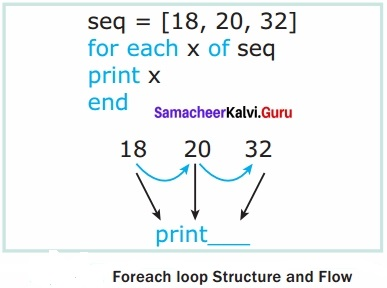Example:
<?php
\$Student_name = array(“Magilan”, “Iniyan”,
“Nilani”, “Sibi”, “Shini”);
foreach (SStudent_name as \$value)
{
echo “Svalue <br>”;
}
?>

Question 3.
Explain the process Do while loop?
Do While Loop:
Do while loop always run the statement inside of the loop block at the first time execution. Then it is checking the condition whether true or false. It executes the loop, if the specified condition is true.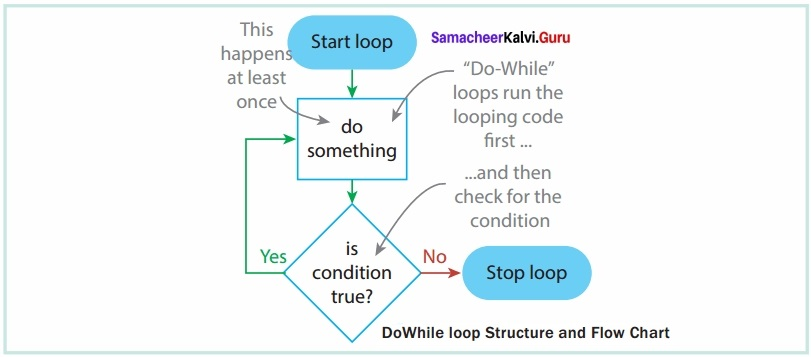Syntax:
do
{
code to be executed;
}
while (condition is true);
Example:
<?php
\$Student_count = 10;
\$student_number=1;
do
{
echo “The student number is: \$student_number<br>”;
\$ student_number++;
}
while(\$student_number<= \$Student_count)
?>

Question 4.
Explain concepts of for loop with example?
For Loop:
For loop is an important functional looping system which is used for iteration logics when the programmer know in advance how many times the loop should run.

Syntax:
for Unit counter; test counter; increment counter)
{
code to be executed;
}

Parameters:

1. init counter: Initialize the loop initial counter value
2. Test counter: Evaluated for every iteration of the loop. If it evaluates to TRUE, the loop continues. If it evaluates to FALSE, the loop ends.
3. Increment counter: Increases the loop counter value.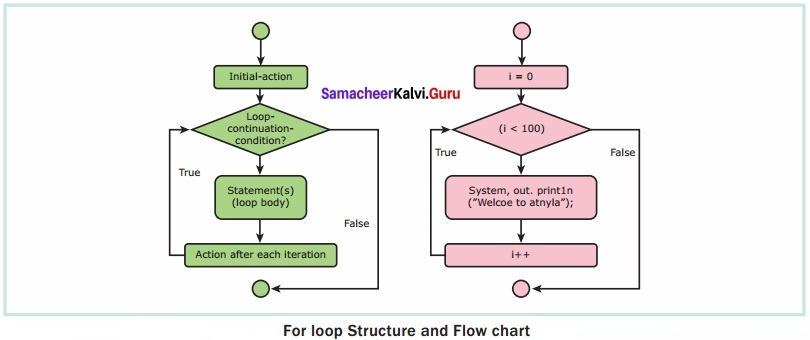Example:
<?php
for (\$i = 0; \$i<= 10; \$i++)
{
echo “The number is: \$i<br>”;
}
?>

Question 5.
Explain array concepts in Looping Structure?
Foreach Loop:
for each loop is exclusively available in PHP. It works only with arrays. The loop iteration deepens on each KEY Value pair in the Array. For each, loop iteration the value of the current array element is assigned to \$value variable and the array pointer is shifted by one, until it reaches the end of the array element.Syntax:
for each (\$array as Svalue)
{
code to be executed;
}
The for each construct provides an easy way to iterate over arrays, foreach works only on arrays Foreach loop Structure and Flow and objects, and will issue an error when you try to use it on a variable with a different data type or an uninitialized variable.
Example:
<?php
\$Student_name = array(“Magilan”, “Iniyan”,
“Nilani”, “Sibi”, “Shini”);
foreach (SStudentname as Svalue) {
echo “Svalue <br>”;
}
?>

### Samacheer Kalvi 12th Computer Applications Solutions Looping Structure Additional Question and Answer

Question 1.
………………………….. structures are useful for writing iteration logics.
Looping

Question 2.
Which of the following is an exit check loop?
a) for each
b) for
c) while
d) do. .while
d) do. .while

Question 3.
Find the true statement from the following.
(a) Init counter initialises the loop
(b) Increment counter initialises the loop
(a) Init counter initialises the loop

Question 4.
……………… loops execute a block of code a specified number of times.
a) foreach
b) for
c) while
d) do..while
b) for

Question 5.
How many segments are there in a for loop?
(a) 1
(b) 2
(c) 3
(d) 4
(c) 3

Question 6.
Pick the odd one out related to the parameters of the for loop?
(a) init
(b) test
(c) text
(d) Increment
(c) text

Question 7.
How many types of loops are there?
(a) 1
(b) 2
(c) 3
(d) 4
(d) 4

Question 8.
Each segment of the for loop is terminated by
(a) ,
(b) ;
(c) :
(d) .
(b) ;

Question 9.
Which loop is exclusively available in PHP?
(a) For
(b) While
(c) for each
(d) do-while
(c) for each

Question 10.
Which of the following loop works only with arrays?
(a) for
(b) while
(c) for each
(d) do-while
(c) for each

Question 11.
Find the Incorrect statement
(I) For loop works only with arrays
(II) For each loop works only with arrays
(I) For loop works only with arrays

Question 12.
Which one of the following loops has key-value pair?
(a) for
(b) for each
(c) while
(d) do.while
(b) for each

Question 13.
Assertion (A): In each loop, the array pointer is shifted by one.
Reason (R): Whether it reaches the end of the array element
(a) A is True, R is correct But R is not the correct explanation for A
(b) Assertion is True, Reason is False
(c) Assertion is false, Reason is true
(d) Assertion and Reason are correct and R is the correct reason for A.
(d) Assertion and Reason are correct and R is the correct reason for A.

Question 14.
Find the Wrong statement
(a) For each work on arrays and pointers
(b) For each work on arrays
(c) For each work on arrays and objects
(d) For each work on objects
(a) For each work on arrays and pointers

Question 15.
Find the correct statement from the following.
(a) For each can be used for variables with a different data type
(b) For each can be used with an uninitialized variable
(c) For each can be used for objects
(c) For each can be used for objects

Question 16.
Which loop is used for simple iteration objects?
(a) For
(b) For each
(c) While
(d) do..while
(c) While

Question 17.
Which one of the following loops will be executed atleast once?
(a) For
(b) For each
(c) While
(d) do..while
(d) do..while

Question 1.
Write a short note on all the loops

• PHP while loops execute a block of code while the specified condition is true.
• The for loop is used when you know in advance how many times the script should run.
• The for-each loop works only on arrays and is used to loop through each key/value pair in an array.
• do…while – loops through a block of code once, and then repeats the loop as long as the specified condition is true.

Question 2.
Give the flow chart for For Loop?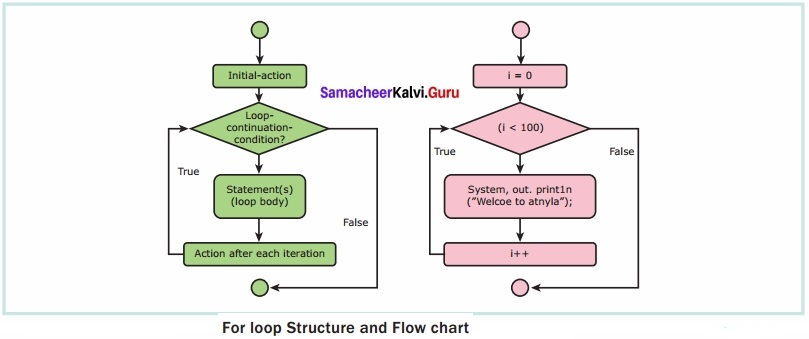Question 3.
Write a php program to print the students name in the array?
Example:
<?php
\$Student_name = array(“Magilan”, “Iniyan”,
“Nilani”, “Sibi”, “Shini”);
foreach (\$Studentname as \$value) { echo “Svalue <br>”;
?>

Question 4.
Give the structure and flow for while loop?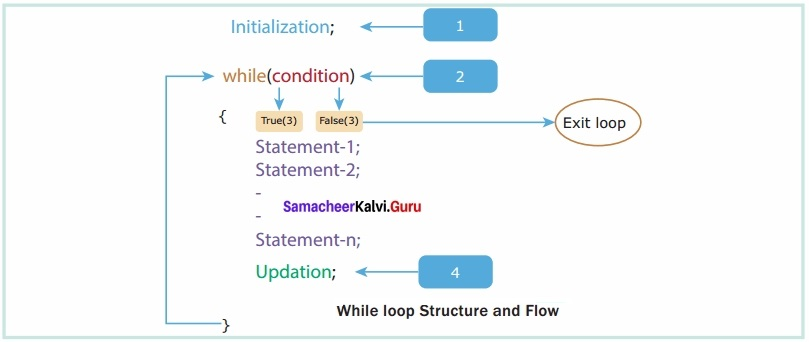Question 5.
Give the structure and flow chart for do while loop?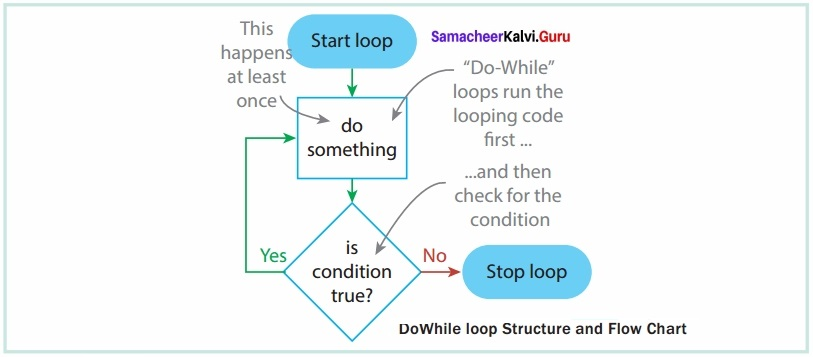Question 6.
Write a php program to print star (*) in a single line?
<?php
for (\$x = 1; \$x < 10;++\$x)
{
print “*\t”;}
?>

III. Explain in detail

Question 1.
Explain While loop?
While Loop:
While loop is an important feature which is used for simple iteration logics. It is checking the condition whether true or false. It executes the loop if the specified condition is true.Syntax:
while (condition is true) {
code to be executed;
}
Example:
<?php
\$Student_count = 10;
\$student_number=1;
while(\$student_number<= \$Student_count)
{
echo “The student number is: \$student_number<br>”;
\$student_number++;
}
?>

## How to Prepare using Samacheer Kalvi 12th Computer Applications Chapter 7 Looping Structure Notes PDF?

Students must prepare for the upcoming exams from Samacheer Kalvi 12th Computer Applications Chapter 7 Looping Structure Notes PDF by following certain essential steps which are provided below.

• Use Samacheer Kalvi 12th Computer Applications Chapter 7 Looping Structure notes by paying attention to facts and ideas.
• Pay attention to the important topics
• Refer TN Board books as well as the books recommended.
• Correctly follow the notes to reduce the number of questions being answered in the exam incorrectly
• Highlight and explain the concepts in details.

## Frequently Asked Questions on Samacheer Kalvi 12th Computer Applications Chapter 7 Looping Structure Notes

#### How to use Samacheer Kalvi 12th Computer Applications Chapter 7 Looping Structure Notes for preparation??

Read TN Board thoroughly, make separate notes for points you forget, formulae, reactions, diagrams. Highlight important points in the book itself and make use of the space provided in the margin to jot down other important points on the same topic from different sources.

#### How to make notes for Samacheer Kalvi 12th Computer Applications Chapter 7 Looping Structure exam?

Read from hand-made notes prepared after understanding concepts, refrain from replicating from the textbook. Use highlighters for important points. Revise from these notes regularly and formulate your own tricks, shortcuts and mnemonics, mappings etc.
Share: